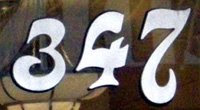## Wednesday, April 29, 2009

### 347

347 is a prime number.

347 is the smallest prime such that the product of its digits is equal to 2 times the number of digits times the sum of digits: 3 x 4 x 7 = 2(3 x (3 + 4 + 7).

347 is the largest three-digit prime that remains prime when 2 is added to any of its digits: 547, 367, and 349 are primes.

347 and 349 form a twin prime pair.Dracula's fictional London house was located at
347 Piccadilly, according to Leslie S. Klinger's book The New Annotated Dracula.

Source:
Prime Curios!